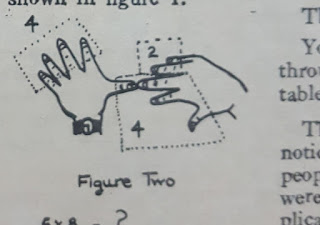# TALES OF A GRANDPA : FINGERS by Dr.Khin Maung Win (Maths)

MATHEMATICS 10

TALES OF A GRANDPA : FINGERS

My children loved listening to stories told by their grandpa , my father -in – law.Here is one of his tales which runs as follows .

In the olden days , men could only learn multiplication tables for 2,3,4 and 5 .In order to multiply by 6,7,8,9 they used their fingers.What they did to multiply by six through nine is as follows.

Beginning with the thumb , let each finger on both hands stand for a number six through ten as shown in figure one .figure one

To multiply, put the tips of the two fingers representing the required numbers together.In figure two, we are multiplying six by eight.figure two

We multiply the number of fingers that are touching as well as those below by ten.That gives 4 × 10 = 40 .

Next we multiply the number of fingers on the left and those on the right which lie above the touching fingers. That gives 4 ×2 = 8 .

Add what you obtained. That gives 40 + 8 = 48

Now 6 × 8= 48figure three

In figure three we are multiplying eight by seven .